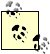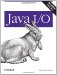# Floating-Point Numbers

Java understands two floating-point number formats, both specified by the IEEE 754 standard. Floats are stored in 4 bytes with a 1-bit sign, a 24-bit mantissa, and an 8-bit exponent. Float values range from 1.40129846432481707 x 10-45 to 3.40282346638528860 x 1038, either positive or negative. Doubles take up 8 bytes with a 1-bit sign, 53-bit mantissa, and 11-bit exponent. This gives them a range of 4.94065645841246544 x 10-324 to 1.79769313486231570 x 10308, either positive or negative. Both floats and doubles also have representations of positive and negative zero, positive and negative infinity, and not a number (NaN).Astute readers will notice that the number of bits given for floats and doubles adds up to 33 and 65 bits, respectivelyone too many for the width of the number. The first bit of the mantissa of a nonzero number is assumed to be 1. With this trick, it is unnecessary to include the first bit of the mantissa. Thus, an extra bit of precision is gained for free.

These formats are supported by most modern RISC architectures and by all current X86 processors. Nowadays the only chips that don't natively support this format are a few embedded processors.

The DataInputStream class reads and the DataOutputStream class writes floating-point numbers of either 4 or 8 bytes in length, as specified in the IEEE 754 standard. They do not support the 10-byte and longer long double, extended double, and double double formats supported by some architectures and compilers. If you have to read floating-point data written in some format other than basic IEEE 754 float and double, you'll need to write your own class to convert the format to 4- or 8-byte IEEE 754.

8.3.1. Writing Floating-Point Numbers

Two methods in the DataOutputStream class write floating-point numbers, writeFloat( ) and writeDouble( ) :

```public final void writeFloat(float f) throws IOException
public final void writeDouble(double d) throws IOException
```

Both of these methods throw an IOException if something goes wrong with the underlying stream. Otherwise, they're fairly innocuous and can convert any float or double to bytes and write it on the underlying stream.

Example 8-3 fills a file called roots.dat with the square roots of the numbers 0 to 1000. First, a FileOutputStream is opened to roots.dat. This stream is chained to a DataOutputStream, whose writeDouble( ) method writes the data into the file.

Example 8-3. Writing doubles with a DataOutputStream

 ```import java.io.*; public class RootsFile { public static void main(String[] args) throws IOException { DataOutputStream dout = null; try { FileOutputStream fout = new FileOutputStream("roots.dat"); dout = new DataOutputStream(fout); for (int i = 0; i <= 1000; i++) { dout.writeDouble(Math.sqrt(i)); } dout.flush( ); dout.close( ); } finally { if (dout != null) dout.close( ); } } } ```

```public final float readFloat( ) throws IOException
public final double readDouble( ) throws IOException
```

The readFloat( ) method reads 4 bytes, converts the data into a float, and returns it. The readDouble( ) method reads 8 bytes, converts the data into a double, and returns that. Both methods throw an EOFException if they can't read enough bytes. In this case, data may be lost without careful (and usually unnecessary) marking and resetting.

Example 8-4 reads a file specified on the command line and prints its contents interpreted as doubles.

 ```import java.io.*; public class DoubleReader { public static void main(String[] args) throws IOException { DataInputStream din = null; try { FileInputStream fin = new FileInputStream(args); System.out.println("-----------" + args + "-----------"); din = new DataInputStream(fin); while (true) { int theNumber = din.readDouble( ); System.out.println(theNumber); } // end while } // end try catch (EOFException ex) { // normal termination din.close( ); } catch (IOException ex) { // abnormal termination System.err.println(ex); } } // end main } // end DoubleReader ```

Here are the first few lines produced when this program is used to read the output of Example 8-4, RootsFile. You may recognize this output as the square roots of the integers between 0 and 9.

```\$ java DoubleReader roots.dat
-----------roots.dat-----------
0.0
1.0
1.4142135623730951
1.7320508075688772
2.0
2.23606797749979
2.449489742783178
2.6457513110645907
2.8284271247461903
3.0
...
```Java I/O
ISBN: 0596527500
EAN: 2147483647
Year: 2004
Pages: 244